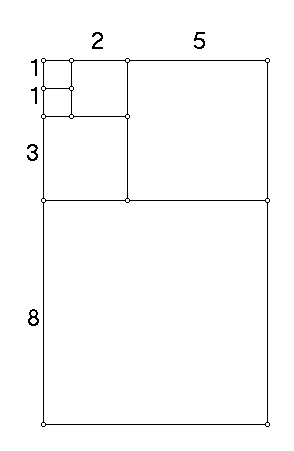Math 240                       Proof without Words #3
M. Flashman

The figure below contains information that can be used to explain why the following statement is true:

If

F 1 = F 2  = 1

and

F n+2 = F n+1 +  F n

then

(F 1 )2 + (F 2 )2 + ... +(F n )2 = (F n ) * (F n+1 )

Fn is called the nth Fibonacci number.
Give a written explanation based on the figure for the truth of the statement.